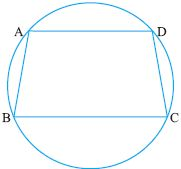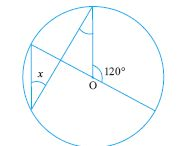Email us to get an instant 20% discount on highly effective K-12 Math & English kwizNET Programs!

#### Online Quiz (WorksheetABCD)

Questions Per Quiz = 2 4 6 8 10

### High School Mathematics - 28.6 Circles - Theorems

 Statement: If two arcs are congruent, then their chords are equal. Given: Arc ASB = Arc CTD To prove: AB = CDProof: OA = OlC, OB = OlD (radii) Angle AOB = Angle COlD (given that the arcs are congruent) Hence by SAS Postulate on congruence AOB is congruent to COlD Hence AB = CD Statement: There is only one circle passing through three non collinear points.Example: In the given figure prove that AB = CDSolution: Arc BAD congruent Arc CDA Hence Arc BAD - Arc AD = Arc CAD - Arc AD Arc BA is congruent Arc CD Hence AB = CD Directions: Solve the following.
 Q 1: Two congruent circles intersect each other at points P and Q, a line through P meets the circles in A and B. Prove that QA = QBAnswer: Q 2: BC is a chord of circle with centre O. A is a point on the major arc BC as shown, prove that angBAC + angOBC = 90.Answer: Q 3: D is the midpoint of side BC of isosceles triangle ABC with AB = AC. Prove that the circle drawn witrh either of the equal sides as diameter pass through D.Answer: Q 4: AC and BD are chords of a circle that bisect each other. Prove that AC and BD are diameters.Answer: Q 5: BC is a chord of circle with centre O. A is a point on the minor arc BC as shown, prove that angBAC - angOBC = 90.Answer: Q 6: Two diameters of a circle intersect each other at right angles. Prove that the quadrilateral formed by joining their end points is a square.Answer: Q 7: Find angle x35o90o55o Q 8: Find the missing angle.35o55o30o Question 9: This question is available to subscribers only! Question 10: This question is available to subscribers only!

#### Subscription to kwizNET Learning System offers the following benefits:

• Unrestricted access to grade appropriate lessons, quizzes, & printable worksheets
• Instant scoring of online quizzes
• Progress tracking and award certificates to keep your student motivated
• Unlimited practice with auto-generated 'WIZ MATH' quizzes